GFG App
Open AppBrowser
Continue

In case of any two variables, the relation between these two variables can be drawn by constructing the table of values if the rule for that relation is mentioned. At least two points coordinates should be known to plot a straight line graph. These points must fit the rule and such graphs when drawn are termed as Linear graphs.

### Linear graphs

A line graph having an unbroken line is called a linear graph. To draw the unbroken line we need to locate some points on graph sheet.

Let’s make graph having x-coordinate and y-coordinate.

Suppose you go to an auditorium and search for your reserved seat. You need to know two numbers, the row number and the seat number. This is the basic method for fixing a point in a plane.

How the location on the board can be described t?

Drawing a point on board namely A1, A2, A3, …., AN measuring these points from the left edge of the board and it is found to be 45 cm. Now we can say that A1 is 45 cm from left edge and 150 cm from the bottom edge.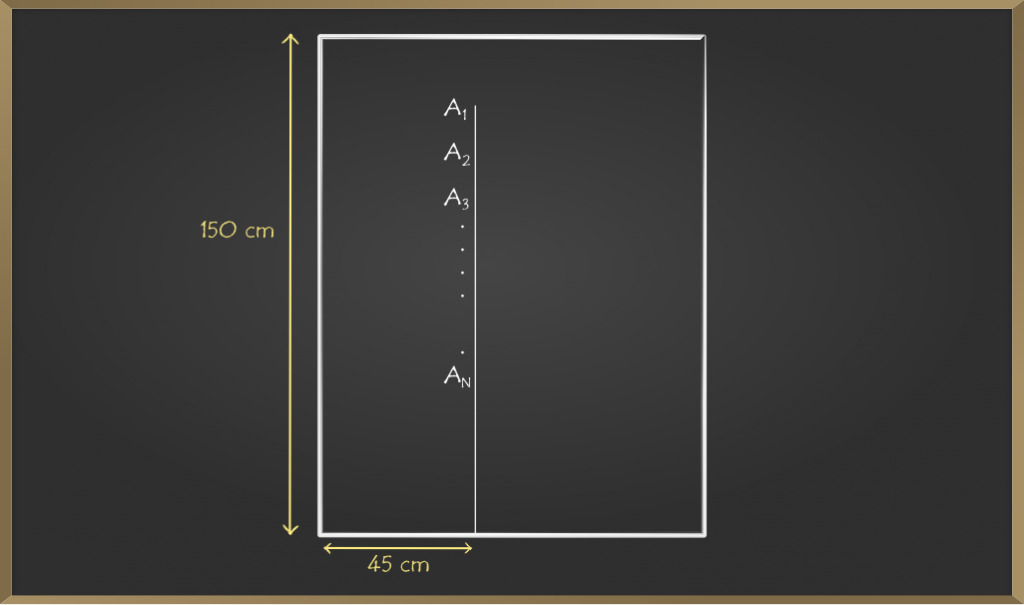### Sample Problems on Linear Graphs

Problem 1: Plot (3, 4) on the graph

Solution:

As in graph points are denoted in the form of (x, y)

So, on comparing the points:

x = 3 and y = 4.

First, draw x = 3 moves in the forward direction to x = 3.

Now, draw y = 4 moves in the upward direction to (3, 4).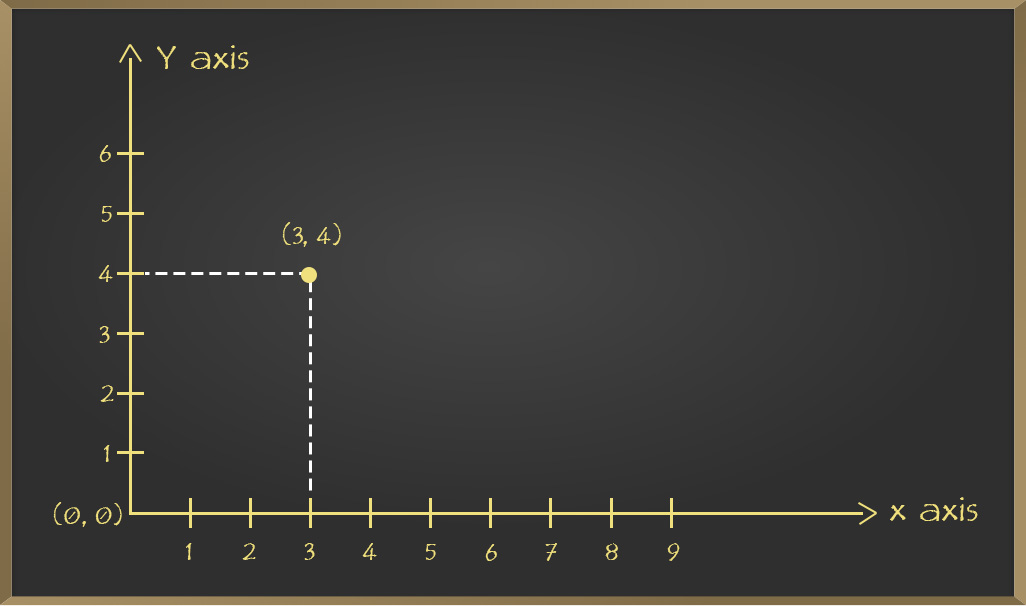Problem 2: Locate the given points on the graph.

1. (1, 2)
2. (2, 8)
3. (4, 2)

Solution:

For x = 1 and y = 2. Starting from (0, 0) origin we move x = 1 direction forward and from there we move y = 2 direction upward then finally reaching state is our point.

Similarly, plot the rest of the two points on Graph.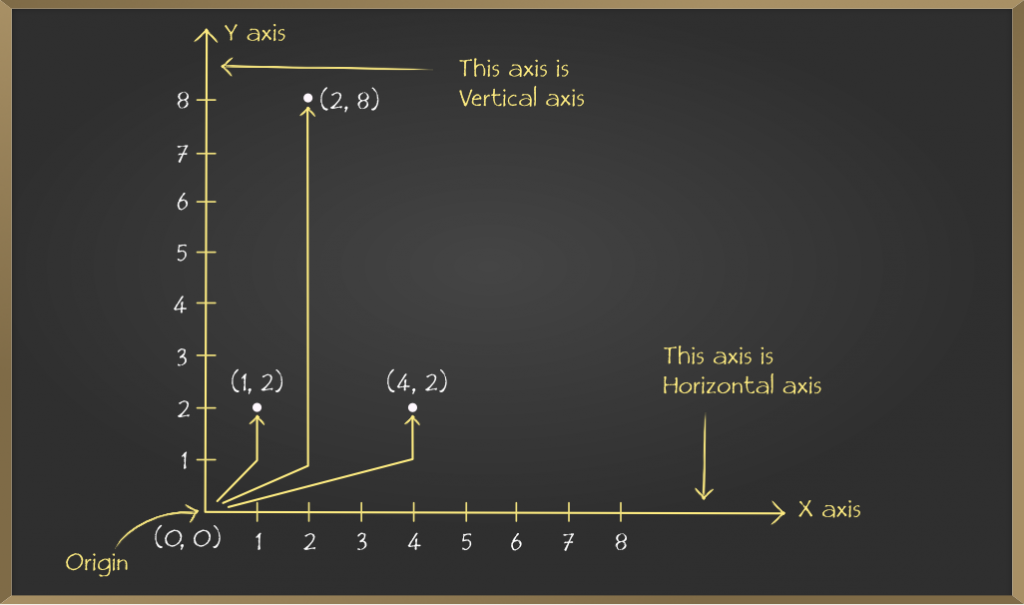Problem 3: Plot the following points and verify if they lie on the line.

1. (0, 1), (0, 2), (0, 4), (0, 3)
2. (1, 1), (2, 2), (3, 3), (4, 4), (5, 5)

Solution:

1. Plotting (0, 1), (0, 2), (0, 4), (0, 3) on graph

We can draw every coordinate on graph sheet as follows .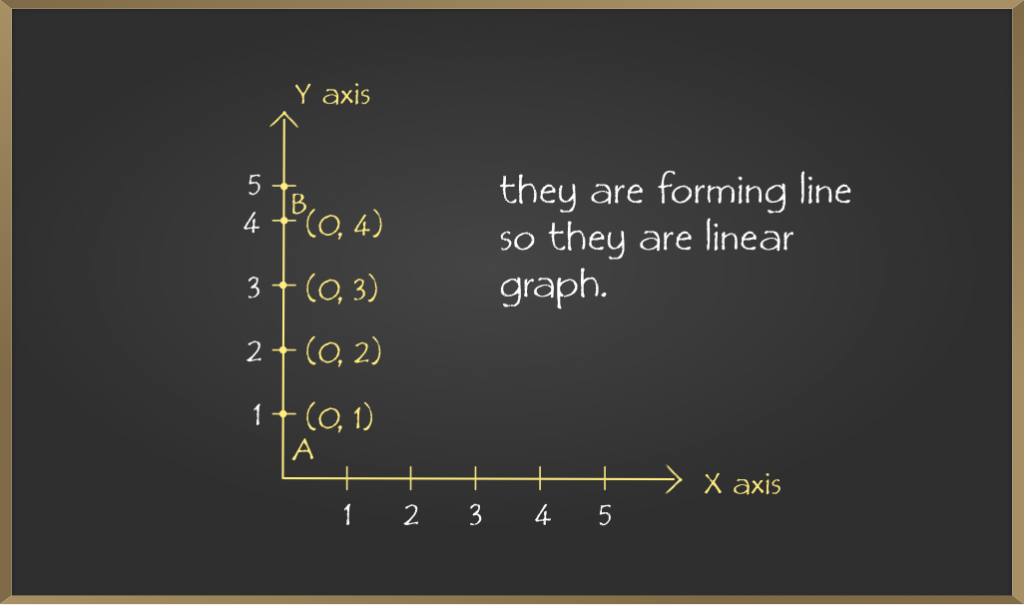Here it forms a line after joining all the points.

2. Plotting (1, 1), (2, 2), (3, 3), (4, 4), (5, 5) on graph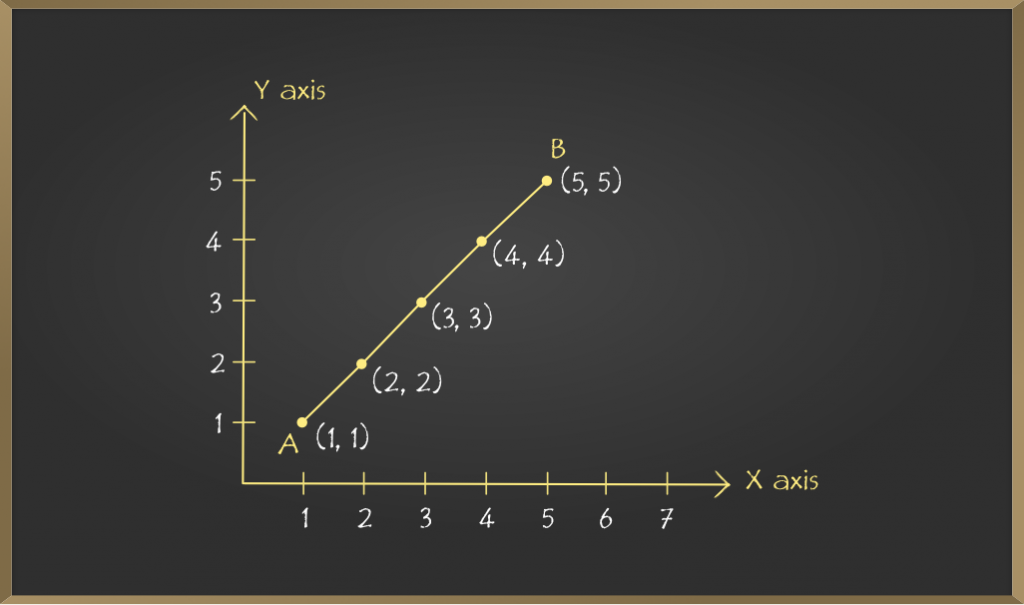Note: In each of the above cases, graph obtained by joining the plotted points is a line. Such graphs are called linear graphs.

### Application of Linear Graphs

In everyday life, we observe variations in the value of different quantities, or we can say that the more we use the facility the more we have to pay for it. for e.g. If the more electricity we consumed then we will have to pay the bill more and vice versa. So one quantity affects the other quantity. We can say that quantity of electricity is the independent variable and the amount of bill is the dependent variable. These relations we can show with graphs.

Problem 1: Amit can ride a bike with a constant speed of 30 km/hour. Draw a time-distance graph for this situation and find it

(i) Time taken by Amit to ride 75 km.

(ii) Distance covered by Amit in 3.5 hours.

Solution:

The table so formed is:

Consider the Scale:

Horizontal: 2 units = 1 hour

Vertical: 1 unit = 10 km

Mark time on horizontal axis.

Mark distance on vertical axis

Plot points: (1, 30), (2, 60), (3, 90), (4, 120)

On joining the points the required linear graph is obtained.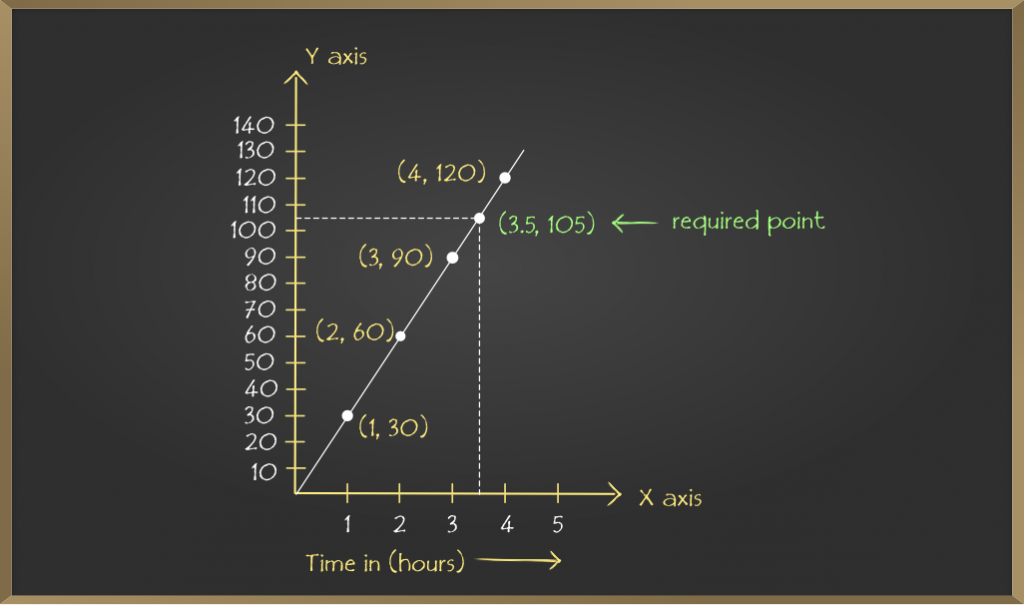(i) From the above graph it is concluded that on the vertical axis corresponding to the distance 75 km, the time is 2.5 hours on the horizontal axis. Thus, 2.5 hours are needed to cover 75 km.

(ii) Similarly, on the horizontal axis corresponding to time 3.5 hours, the distance covered is 105 km on the vertical axis.

Problem 2: A public bank provides 10% interest on the deposits by senior citizens. Plot a graph representing the relation between the deposited amount and the interest earned by senior citizens and state the following things from the graph so plotted:

(i) The interest earned annually for an investment of 450.

(ii) The amount of money deposited to earn the interest of ₹ 35.

Solution:

Consider the Scale:

Horizontal: 1 unit = ₹ 10

Vertical: 1 unit = ₹ 100

Mark interest earned on horizontal axis.

Mark amount deposited on vertical axis.

Plot the points: (10, 100), (30, 300), (50, 500), (70, 700) and (90, 900) on the graph.

On joining the points the required linear graph is obtained.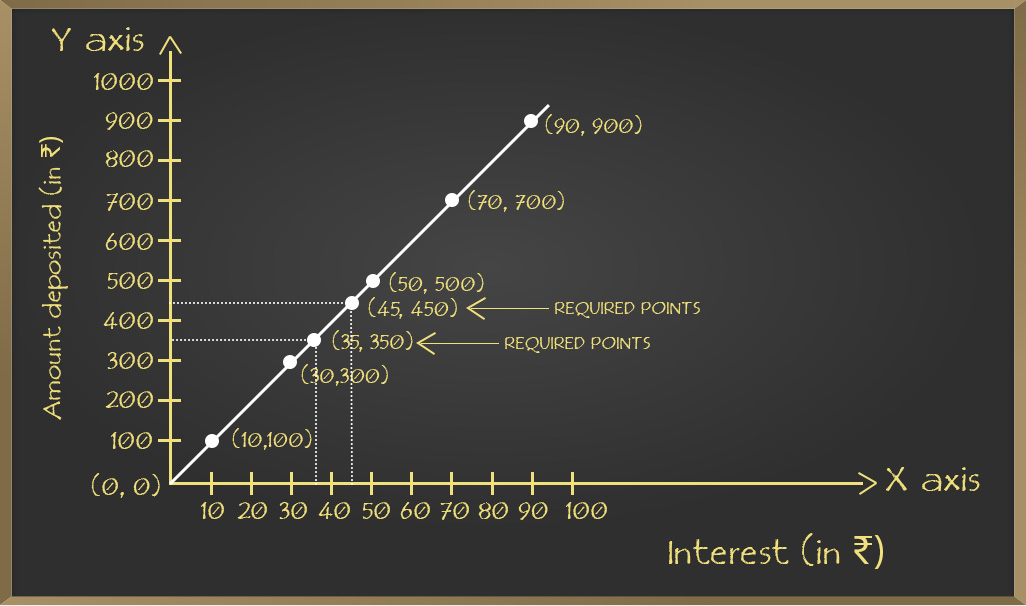(i) From the above graph it is concluded that on the vertical axis corresponding to the amount deposited ₹ 450, the interest earned is ₹ 45 on the horizontal axis. Thus, ₹ 45 are earned on the deposit of ₹ 450.

(ii) Similarly, on the horizontal axis corresponding to interest earned 35%, the amount deposited is ₹ 350 on the vertical axis.

My Personal Notes arrow_drop_up